31.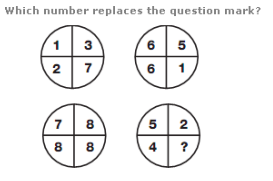A. 7 B. 8 C. 9 D. 6

Explanation: :

Numbers in the lower left circle equal the sum of the numbers in corresponding segments of the top two circles, and numbers in the lower right circle equal the difference of the numbers in corresponding segments of the top two circles.

32.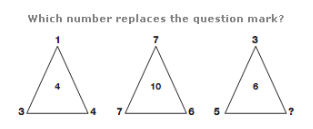A. 5 B. 4 C. 1 D. 3

Explanation: :

In each triangle, the central number equals the sum of the three outer num

33.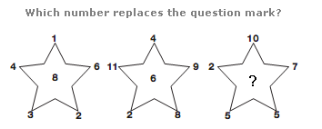A. 6 B. 7 C. 9 D. 5

Explanation: :

In each star, the central number equals the difference between the sum of the even numbers and the sum of the odd numbers from the points of the star.

34.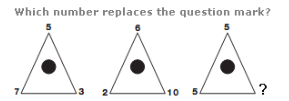A. 8 B. 9 C. 11 D. 5

Explanation: :

In each triangle, the number at the apex of the triangle equals the average of the two numbers at the bottom.

35.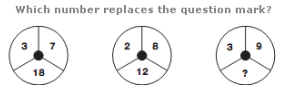A. 33 B. 44 C. 22 D. 55